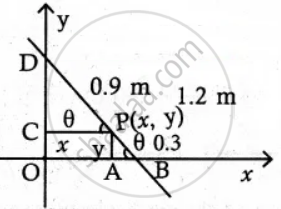Tamil Nadu Board of Secondary EducationHSC Arts Class 12th

# A rod of length 1 2. m moves with its ends always touching the coordinate axes. The locus of a point P on the rod, which is 0 3. m from the end in contact with x -axis is an ellipse. Find the - Mathematics

Sum

A rod of length 1 2. m moves with its ends always touching the coordinate axes. The locus of a point P on the rod, which is 0 3. m from the end in contact with x-axis is an ellipse. Find the eccentricity

#### SolutionLength of rod BD = 1.2 m

Let P(x, y) be any point on the Rod such
that PB = 0.3 m

∴ PD = 1.2 – 0.3

= 0.9 m

Let ΔPAB and ΔPCD are similar triangles

In ΔPAB sin θ = y/(0.3)

In ΔPCB cos θ = y/(0.9)

We know that sin2θ + cos2θ = 1

y^2/(0.3^2) + x^2/(0.9^2) = 1

x^2/(0.9^2) + y^2/(0.3^2) = 1

a2 = 0.92

b2 = 0.32

Eccentricity e = sqrt(1 - "b"^2/"a"^2)

= sqrt(1 - 0.3^2/0.9^2)

= sqrt(1 - (1/3)^2

= sqrt(1 - 1/9)

= sqrt(8/9)

= (2sqrt(2))/3

Concept: Real Life Applications of Conics
Is there an error in this question or solution?

#### APPEARS IN

Tamil Nadu Board Samacheer Kalvi Class 12th Mathematics Volume 1 and 2 Answers Guide
Chapter 5 Two Dimensional Analytical Geometry-II
Exercise 5.5 | Q 7 | Page 215
Share